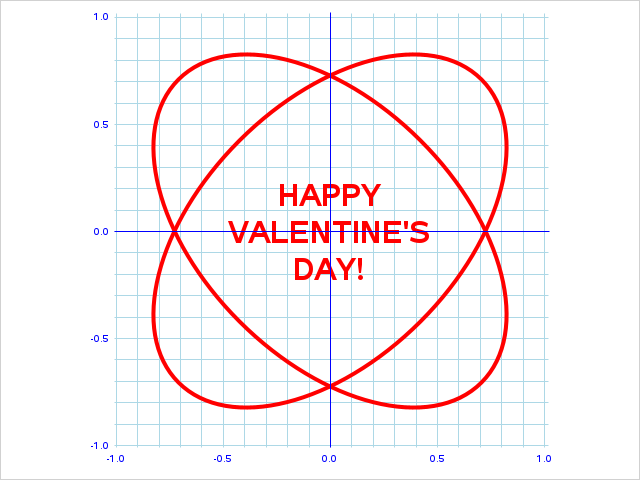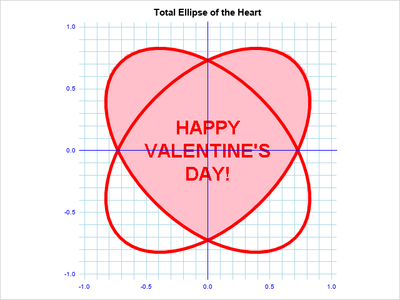## Fun w/SAS ODS Graphics: Total Ellipse of the HeartInspired by Amanda Newton's clever The Heart of Mathematics (Valentine's Day Heart), here's how to turn two ellipses into two hearts. Happy Valentine's Day!

``````* Fun w/SAS ODS Graphics: Total Ellipse of the Heart (Two Tilted Ellipses = Two Hearts)
SAS implementation of Amanda Newton's clever "The Heart of Mathematics (Valentine's Day Heart)"
http://jwilson.coe.uga.edu/EMAT6680Su10/Newton/emat6690/Hearts/Hearts.html;

data heart;                                                                    * Display "Happy Valentine's Day at (0,0);
retain x y 0 msg "HAPPY*VALENTINE'S*DAY!";

proc sgplot data=heart noborder nowall noautolegend aspect=1;                  * Plot two intersecting ellipses at +/- 45 degrees to make upright and upside-down hearts;
ellipseparm semimajor=1 semiminor=.6 / slope=1 xorigin=0 yorigin=0 lineattrs=(color=red)  lineattrs=(thickness=3pt) nofill fillattrs=(color=cxC3540C); * Ellipse 1, angled at 45 degrees;
ellipseparm semimajor=1 semiminor=.6 / slope=-1 xorigin=0 yorigin=0 lineattrs=(color=red) lineattrs=(thickness=3pt) nofill fillattrs=(color=cxC3540C); * Ellipse 2, angled at -45 degrees;
text x=x y=y text=msg / textattrs=(size=22pt weight=bold color=red) splitchar='*' splitpolicy=splitalways contributeoffsets=none; * "Happy Valentine's Day!" message;
xaxis display=(nolabel noline noticks) values=(-1 to 1 by .5) valueattrs=(size=7pt color=blue) grid gridattrs=(color=lightblue) minorgrid minorgridattrs=(color=lightblue) minorcount=4;
yaxis display=(nolabel noline noticks) values=(-1 to 1 by .5) valueattrs=(size=7pt color=blue) grid gridattrs=(color=lightblue) minorgrid minorgridattrs=(color=lightblue) minorcount=4;
refline 0 / axis=x lineattrs=(color=blue thickness=1pt); * Reference lines thru (0,0);
refline 0 / axis=y lineattrs=(color=blue thickness=1pt); * Reference lines thru (0,0);
run;``````
1 ACCEPTED SOLUTION

Accepted Solutions

## Re: Fun w/SAS ODS Graphics: Total Ellipse of the Heart

Cute, as usual.

You use a different scaling than newton's article, so I just eyeballed the following enhancement to your graph. I think adding fill helps to see the heart inside of the ellipses:

``````
data heart;                                                                    * Display "Happy Valentine's Day at (0,0);
retain x y 0 msg "HAPPY*VALENTINE'S*DAY!";
do hx = -1.2 to 1.2 by 0.025;
do hy = -1 to 1.2 by 0.025;
if (hx**2 + hy**2 - hy*abs(hx) < 1) then do;
xx = 0.71*hx;
yy = 0.71*hy;
output;
end;
end;
end;
drop hx hy;
run;

title "Total Ellipse of the Heart";
proc sgplot data=heart noborder nowall noautolegend aspect=1;                  * Plot two intersecting ellipses at +/- 45 degrees to make upright and upside-down hearts;
scatter x=xx y=yy / markerattrs=(color=pink symbol=SquareFilled size=9);
ellipseparm semimajor=1 semiminor=.6 / slope=1 xorigin=0 yorigin=0 lineattrs=(color=red)  lineattrs=(thickness=4pt) nofill fillattrs=(color=cxC3540C); * Ellipse 1, angled at 45 degrees;
ellipseparm semimajor=1 semiminor=.6 / slope=-1 xorigin=0 yorigin=0 lineattrs=(color=red) lineattrs=(thickness=4pt) nofill fillattrs=(color=cxC3540C); * Ellipse 2, angled at -45 degrees;
text x=x y=y text=msg / textattrs=(size=22pt weight=bold color=red) splitchar='*' splitpolicy=splitalways contributeoffsets=none; * "Happy Valentine's Day!" message;
xaxis display=(nolabel noline noticks) values=(-1 to 1 by .5) valueattrs=(size=7pt color=blue) grid gridattrs=(color=lightblue) minorgrid minorgridattrs=(color=lightblue) minorcount=4;
yaxis display=(nolabel noline noticks) values=(-1 to 1 by .5) valueattrs=(size=7pt color=blue) grid gridattrs=(color=lightblue) minorgrid minorgridattrs=(color=lightblue) minorcount=4;
refline 0 / axis=x lineattrs=(color=blue thickness=1pt); * Reference lines thru (0,0);
refline 0 / axis=y lineattrs=(color=blue thickness=1pt); * Reference lines thru (0,0);
run;
``````2 REPLIES 2

## Re: Fun w/SAS ODS Graphics: Total Ellipse of the Heart

Cute, as usual.

You use a different scaling than newton's article, so I just eyeballed the following enhancement to your graph. I think adding fill helps to see the heart inside of the ellipses:

``````
data heart;                                                                    * Display "Happy Valentine's Day at (0,0);
retain x y 0 msg "HAPPY*VALENTINE'S*DAY!";
do hx = -1.2 to 1.2 by 0.025;
do hy = -1 to 1.2 by 0.025;
if (hx**2 + hy**2 - hy*abs(hx) < 1) then do;
xx = 0.71*hx;
yy = 0.71*hy;
output;
end;
end;
end;
drop hx hy;
run;

title "Total Ellipse of the Heart";
proc sgplot data=heart noborder nowall noautolegend aspect=1;                  * Plot two intersecting ellipses at +/- 45 degrees to make upright and upside-down hearts;
scatter x=xx y=yy / markerattrs=(color=pink symbol=SquareFilled size=9);
ellipseparm semimajor=1 semiminor=.6 / slope=1 xorigin=0 yorigin=0 lineattrs=(color=red)  lineattrs=(thickness=4pt) nofill fillattrs=(color=cxC3540C); * Ellipse 1, angled at 45 degrees;
ellipseparm semimajor=1 semiminor=.6 / slope=-1 xorigin=0 yorigin=0 lineattrs=(color=red) lineattrs=(thickness=4pt) nofill fillattrs=(color=cxC3540C); * Ellipse 2, angled at -45 degrees;
text x=x y=y text=msg / textattrs=(size=22pt weight=bold color=red) splitchar='*' splitpolicy=splitalways contributeoffsets=none; * "Happy Valentine's Day!" message;
xaxis display=(nolabel noline noticks) values=(-1 to 1 by .5) valueattrs=(size=7pt color=blue) grid gridattrs=(color=lightblue) minorgrid minorgridattrs=(color=lightblue) minorcount=4;
yaxis display=(nolabel noline noticks) values=(-1 to 1 by .5) valueattrs=(size=7pt color=blue) grid gridattrs=(color=lightblue) minorgrid minorgridattrs=(color=lightblue) minorcount=4;
refline 0 / axis=x lineattrs=(color=blue thickness=1pt); * Reference lines thru (0,0);
refline 0 / axis=y lineattrs=(color=blue thickness=1pt); * Reference lines thru (0,0);
run;
``````## Re: Fun w/SAS ODS Graphics: Total Ellipse of the Heart

Thaks @Rick_SAS  - that is much better!

Discussion stats
• 2 replies
• 502 views
• 5 likes
• 2 in conversation